# garage.tf.policies¶

Policies for TensorFlow-based algorithms.

class CategoricalCNNPolicy(env_spec, filters, strides, padding, name='CategoricalCNNPolicy', hidden_sizes=(32, 32), hidden_nonlinearity=tf.nn.relu, hidden_w_init=tf.initializers.glorot_uniform(seed=deterministic.get_tf_seed_stream()), hidden_b_init=tf.zeros_initializer(), output_nonlinearity=tf.nn.softmax, output_w_init=tf.initializers.glorot_uniform(seed=deterministic.get_tf_seed_stream()), output_b_init=tf.zeros_initializer(), layer_normalization=False)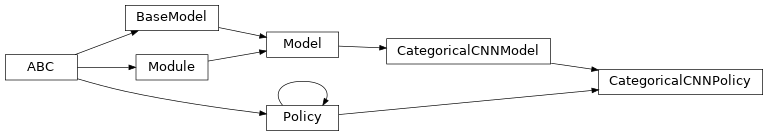CategoricalCNNPolicy.

A policy that contains a CNN and a MLP to make prediction based on a categorical distribution.

It only works with akro.Discrete action space.

Parameters
• env_spec (garage.envs.env_spec.EnvSpec) – Environment specification.

• filters (Tuple[Tuple[int, Tuple[int, int]], ..]) – Number and dimension of filters. For example, ((3, (3, 5)), (32, (3, 3))) means there are two convolutional layers. The filter for the first layer have 3 channels and its shape is (3 x 5), while the filter for the second layer have 32 channels and its shape is (3 x 3).

• strides (tuple[int]) – The stride of the sliding window. For example, (1, 2) means there are two convolutional layers. The stride of the filter for first layer is 1 and that of the second layer is 2.

• padding (str) – The type of padding algorithm to use, either ‘SAME’ or ‘VALID’.

• name (str) – Policy name, also the variable scope of the policy.

• hidden_sizes (list[int]) – Output dimension of dense layer(s). For example, (32, 32) means the MLP of this policy consists of two hidden layers, each with 32 hidden units.

• hidden_nonlinearity (callable) – Activation function for intermediate dense layer(s). It should return a tf.Tensor. Set it to None to maintain a linear activation.

• hidden_w_init (callable) – Initializer function for the weight of intermediate dense layer(s). The function should return a tf.Tensor.

• hidden_b_init (callable) – Initializer function for the bias of intermediate dense layer(s). The function should return a tf.Tensor.

• output_nonlinearity (callable) – Activation function for output dense layer. It should return a tf.Tensor. Set it to None to maintain a linear activation.

• output_w_init (callable) – Initializer function for the weight of output dense layer(s). The function should return a tf.Tensor.

• output_b_init (callable) – Initializer function for the bias of output dense layer(s). The function should return a tf.Tensor.

• layer_normalization (bool) – Bool for using layer normalization or not.

property input_dim(self)

int: Dimension of the policy input.

get_action(self, observation)

Return a single action.

Parameters

observation (numpy.ndarray) – Observations.

Returns

Action given input observation. dict(numpy.ndarray): Distribution parameters.

Return type

int

get_actions(self, observations)

Return multiple actions.

Parameters

observations (numpy.ndarray) – Observations.

Returns

Actions given input observations. dict(numpy.ndarray): Distribution parameters.

Return type

list[int]

property env_spec(self)

Policy environment specification.

Returns

Environment specification.

Return type

garage.EnvSpec

clone(self, name)

Return a clone of the policy.

It copies the configuration of the primitive and also the parameters.

Parameters

name (str) – Name of the newly created policy. It has to be different from source policy if cloned under the same computational graph.

Returns

Newly cloned policy.

Return type

garage.tf.policies.CategoricalCNNPolicy

network_output_spec(self)

Network output spec.

Returns

Name of the model outputs, in order.

Return type

list[str]

build(self, *inputs, name=None)

Build a Network with the given input(s).

* Do not call tf.global_variable_initializers() after building a model as it will reassign random weights to the model. The parameters inside a model will be initialized when calling build(). *

It uses the same, fixed variable scope for all Networks, to ensure parameter sharing. Different Networks must have an unique name.

Parameters
• inputs (list[tf.Tensor]) – Tensor input(s), recommended to be positional arguments, for example, def build(self, state_input, action_input, name=None).

• name (str) – Name of the model, which is also the name scope of the model.

Raises

ValueError – When a Network with the same name is already built.

Returns

Output tensors of the model with the given

inputs.

Return type

list[tf.Tensor]

network_input_spec(self)

Network input spec.

Returns

List of key(str) for the network inputs.

Return type

list[str]

property parameters(self)

Parameters of the model.

Returns

Parameters

Return type

np.ndarray

property name(self)

Name (str) of the model.

This is also the variable scope of the model.

Returns

Name of the model.

Return type

str

property input(self)

Default input of the model.

When the model is built the first time, by default it creates the ‘default’ network. This property creates a reference to the input of the network.

Returns

Default input of the model.

Return type

tf.Tensor

property output(self)

Default output of the model.

When the model is built the first time, by default it creates the ‘default’ network. This property creates a reference to the output of the network.

Returns

Default output of the model.

Return type

tf.Tensor

property inputs(self)

Default inputs of the model.

When the model is built the first time, by default it creates the ‘default’ network. This property creates a reference to the inputs of the network.

Returns

Default inputs of the model.

Return type

list[tf.Tensor]

property outputs(self)

Default outputs of the model.

When the model is built the first time, by default it creates the ‘default’ network. This property creates a reference to the outputs of the network.

Returns

Default outputs of the model.

Return type

list[tf.Tensor]

reset(self, do_resets=None)

Reset the module.

This is effective only to recurrent modules. do_resets is effective only to vectoried modules.

For a vectorized modules, do_resets is an array of boolean indicating which internal states to be reset. The length of do_resets should be equal to the length of inputs.

Parameters

do_resets (numpy.ndarray) – Bool array indicating which states to be reset.

property state_info_specs(self)

State info specification.

Returns

keys and shapes for the information related to the

module’s state when taking an action.

Return type

List[str]

property state_info_keys(self)

State info keys.

Returns

keys for the information related to the module’s state

when taking an input.

Return type

List[str]

terminate(self)

Clean up operation.

get_trainable_vars(self)

Get trainable variables.

Returns

A list of trainable variables in the current

variable scope.

Return type

List[tf.Variable]

get_global_vars(self)

Get global variables.

Returns

A list of global variables in the current

variable scope.

Return type

List[tf.Variable]

get_regularizable_vars(self)

Get all network weight variables in the current scope.

Returns

A list of network weight variables in the

current variable scope.

Return type

List[tf.Variable]

get_params(self)

Get the trainable variables.

Returns

A list of trainable variables in the current

variable scope.

Return type

List[tf.Variable]

get_param_shapes(self)

Get parameter shapes.

Returns

A list of variable shapes.

Return type

List[tuple]

get_param_values(self)

Get param values.

Returns

Values of the parameters evaluated in

the current session

Return type

np.ndarray

set_param_values(self, param_values)

Set param values.

Parameters

param_values (np.ndarray) – A numpy array of parameter values.

flat_to_params(self, flattened_params)

Unflatten tensors according to their respective shapes.

Parameters

flattened_params (np.ndarray) – A numpy array of flattened params.

Returns

A list of parameters reshaped to the

shapes specified.

Return type

List[np.ndarray]

property observation_space(self)

Observation space.

Returns

The observation space of the environment.

Return type

akro.Space

property action_space(self)

Action space.

Returns

The action space of the environment.

Return type

akro.Space

class CategoricalGRUPolicy(env_spec, name='CategoricalGRUPolicy', hidden_dim=32, hidden_nonlinearity=tf.nn.tanh, hidden_w_init=tf.initializers.glorot_uniform(seed=deterministic.get_tf_seed_stream()), hidden_b_init=tf.zeros_initializer(), recurrent_nonlinearity=tf.nn.sigmoid, recurrent_w_init=tf.initializers.glorot_uniform(seed=deterministic.get_tf_seed_stream()), output_nonlinearity=tf.nn.softmax, output_w_init=tf.initializers.glorot_uniform(seed=deterministic.get_tf_seed_stream()), output_b_init=tf.zeros_initializer(), hidden_state_init=tf.zeros_initializer(), hidden_state_init_trainable=False, state_include_action=True, layer_normalization=False)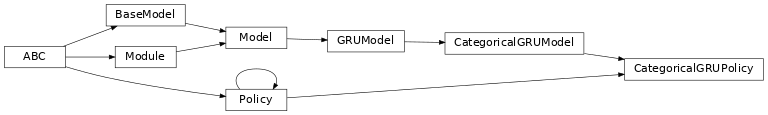Categorical GRU Policy.

A policy represented by a Categorical distribution which is parameterized by a Gated Recurrent Unit (GRU).

It only works with akro.Discrete action space.

Parameters
• env_spec (garage.envs.env_spec.EnvSpec) – Environment specification.

• name (str) – Policy name, also the variable scope.

• hidden_dim (int) – Hidden dimension for LSTM cell.

• hidden_nonlinearity (callable) – Activation function for intermediate dense layer(s). It should return a tf.Tensor. Set it to None to maintain a linear activation.

• hidden_w_init (callable) – Initializer function for the weight of intermediate dense layer(s). The function should return a tf.Tensor.

• hidden_b_init (callable) – Initializer function for the bias of intermediate dense layer(s). The function should return a tf.Tensor.

• recurrent_nonlinearity (callable) – Activation function for recurrent layers. It should return a tf.Tensor. Set it to None to maintain a linear activation.

• recurrent_w_init (callable) – Initializer function for the weight of recurrent layer(s). The function should return a tf.Tensor.

• output_nonlinearity (callable) – Activation function for output dense layer. It should return a tf.Tensor. Set it to None to maintain a linear activation.

• output_w_init (callable) – Initializer function for the weight of output dense layer(s). The function should return a tf.Tensor.

• output_b_init (callable) – Initializer function for the bias of output dense layer(s). The function should return a tf.Tensor.

• hidden_state_init (callable) – Initializer function for the initial hidden state. The functino should return a tf.Tensor.

• hidden_state_init_trainable (bool) – Bool for whether the initial hidden state is trainable.

• state_include_action (bool) – Whether the state includes action. If True, input dimension will be (observation dimension + action dimension).

• layer_normalization (bool) – Bool for using layer normalization or not.

build(self, state_input, name=None)

Build policy.

Parameters
• state_input (tf.Tensor) – State input.

• name (str) – Name of the policy, which is also the name scope.

Returns

Policy distribution. tf.Tensor: Step output, with shape $$(N, S^*)$$. tf.Tensor: Step hidden state, with shape $$(N, S^*)$$. tf.Tensor: Initial hidden state , used to reset the hidden state

when policy resets. Shape: $$(S^*)$$.

Return type

tfp.distributions.OneHotCategorical

property input_dim(self)

int: Dimension of the policy input.

reset(self, do_resets=None)

Reset the policy.

Note

If do_resets is None, it will be by default np.array([True]), which implies the policy will not be “vectorized”, i.e. number of paralle environments for training data sampling = 1.

Parameters

do_resets (numpy.ndarray) – Bool that indicates terminal state(s).

get_action(self, observation)

Return a single action.

Parameters

observation (numpy.ndarray) – Observations.

Returns

Action given input observation. dict(numpy.ndarray): Distribution parameters.

Return type

int

get_actions(self, observations)

Return multiple actions.

Parameters

observations (numpy.ndarray) – Observations.

Returns

Actions given input observations. dict(numpy.ndarray): Distribution parameters.

Return type

list[int]

property env_spec(self)

Policy environment specification.

Returns

Environment specification.

Return type

garage.EnvSpec

property state_info_specs(self)

State info specifcation.

Returns

keys and shapes for the information related to the

policy’s state when taking an action.

Return type

List[str]

clone(self, name)

Return a clone of the policy.

It copies the configuration of the primitive and also the parameters.

Parameters

name (str) – Name of the newly created policy. It has to be different from source policy if cloned under the same computational graph.

Returns

Newly cloned policy.

Return type

garage.tf.policies.CategoricalGRUPolicy

network_output_spec(self)

Network output spec.

Returns

Name of the model outputs, in order.

Return type

list[str]

network_input_spec(self)

Network input spec.

Returns

List of key(str) for the network outputs.

Return type

list[str]

property parameters(self)

Parameters of the model.

Returns

Parameters

Return type

np.ndarray

property name(self)

Name (str) of the model.

This is also the variable scope of the model.

Returns

Name of the model.

Return type

str

property input(self)

Default input of the model.

When the model is built the first time, by default it creates the ‘default’ network. This property creates a reference to the input of the network.

Returns

Default input of the model.

Return type

tf.Tensor

property output(self)

Default output of the model.

When the model is built the first time, by default it creates the ‘default’ network. This property creates a reference to the output of the network.

Returns

Default output of the model.

Return type

tf.Tensor

property inputs(self)

Default inputs of the model.

When the model is built the first time, by default it creates the ‘default’ network. This property creates a reference to the inputs of the network.

Returns

Default inputs of the model.

Return type

list[tf.Tensor]

property outputs(self)

Default outputs of the model.

When the model is built the first time, by default it creates the ‘default’ network. This property creates a reference to the outputs of the network.

Returns

Default outputs of the model.

Return type

list[tf.Tensor]

property state_info_keys(self)

State info keys.

Returns

keys for the information related to the module’s state

when taking an input.

Return type

List[str]

terminate(self)

Clean up operation.

get_trainable_vars(self)

Get trainable variables.

Returns

A list of trainable variables in the current

variable scope.

Return type

List[tf.Variable]

get_global_vars(self)

Get global variables.

Returns

A list of global variables in the current

variable scope.

Return type

List[tf.Variable]

get_regularizable_vars(self)

Get all network weight variables in the current scope.

Returns

A list of network weight variables in the

current variable scope.

Return type

List[tf.Variable]

get_params(self)

Get the trainable variables.

Returns

A list of trainable variables in the current

variable scope.

Return type

List[tf.Variable]

get_param_shapes(self)

Get parameter shapes.

Returns

A list of variable shapes.

Return type

List[tuple]

get_param_values(self)

Get param values.

Returns

Values of the parameters evaluated in

the current session

Return type

np.ndarray

set_param_values(self, param_values)

Set param values.

Parameters

param_values (np.ndarray) – A numpy array of parameter values.

flat_to_params(self, flattened_params)

Unflatten tensors according to their respective shapes.

Parameters

flattened_params (np.ndarray) – A numpy array of flattened params.

Returns

A list of parameters reshaped to the

shapes specified.

Return type

List[np.ndarray]

property observation_space(self)

Observation space.

Returns

The observation space of the environment.

Return type

akro.Space

property action_space(self)

Action space.

Returns

The action space of the environment.

Return type

akro.Space

class CategoricalLSTMPolicy(env_spec, name='CategoricalLSTMPolicy', hidden_dim=32, hidden_nonlinearity=tf.nn.tanh, hidden_w_init=tf.initializers.glorot_uniform(seed=deterministic.get_tf_seed_stream()), hidden_b_init=tf.zeros_initializer(), recurrent_nonlinearity=tf.nn.sigmoid, recurrent_w_init=tf.initializers.glorot_uniform(seed=deterministic.get_tf_seed_stream()), output_nonlinearity=tf.nn.softmax, output_w_init=tf.initializers.glorot_uniform(seed=deterministic.get_tf_seed_stream()), output_b_init=tf.zeros_initializer(), hidden_state_init=tf.zeros_initializer(), hidden_state_init_trainable=False, cell_state_init=tf.zeros_initializer(), cell_state_init_trainable=False, state_include_action=True, forget_bias=True, layer_normalization=False)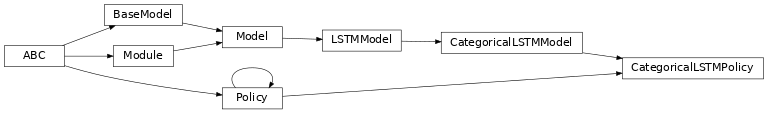Categorical LSTM Policy.

A policy represented by a Categorical distribution which is parameterized by a Long short-term memory (LSTM).

It only works with akro.Discrete action space.

Parameters
• env_spec (garage.envs.env_spec.EnvSpec) – Environment specification.

• name (str) – Policy name, also the variable scope.

• hidden_dim (int) – Hidden dimension for LSTM cell.

• hidden_nonlinearity (callable) – Activation function for intermediate dense layer(s). It should return a tf.Tensor. Set it to None to maintain a linear activation.

• hidden_w_init (callable) – Initializer function for the weight of intermediate dense layer(s). The function should return a tf.Tensor.

• hidden_b_init (callable) – Initializer function for the bias of intermediate dense layer(s). The function should return a tf.Tensor.

• recurrent_nonlinearity (callable) – Activation function for recurrent layers. It should return a tf.Tensor. Set it to None to maintain a linear activation.

• recurrent_w_init (callable) – Initializer function for the weight of recurrent layer(s). The function should return a tf.Tensor.

• output_nonlinearity (callable) – Activation function for output dense layer. It should return a tf.Tensor. Set it to None to maintain a linear activation.

• output_w_init (callable) – Initializer function for the weight of output dense layer(s). The function should return a tf.Tensor.

• output_b_init (callable) – Initializer function for the bias of output dense layer(s). The function should return a tf.Tensor.

• hidden_state_init (callable) – Initializer function for the initial hidden state. The functino should return a tf.Tensor.

• hidden_state_init_trainable (bool) – Bool for whether the initial hidden state is trainable.

• cell_state_init (callable) – Initializer function for the initial cell state. The functino should return a tf.Tensor.

• cell_state_init_trainable (bool) – Bool for whether the initial cell state is trainable.

• state_include_action (bool) – Whether the state includes action. If True, input dimension will be (observation dimension + action dimension).

• forget_bias (bool) – If True, add 1 to the bias of the forget gate at initialization. It’s used to reduce the scale of forgetting at the beginning of the training.

• layer_normalization (bool) – Bool for using layer normalization or not.

build(self, state_input, name=None)

Build policy.

Parameters
• state_input (tf.Tensor) – State input.

• name (str) – Name of the policy, which is also the name scope.

Returns

Policy distribution. tf.Tensor: Step output, with shape $$(N, S^*)$$ tf.Tensor: Step hidden state, with shape $$(N, S^*)$$ tf.Tensor: Step cell state, with shape $$(N, S^*)$$ tf.Tensor: Initial hidden state, used to reset the hidden state

when policy resets. Shape: $$(S^*)$$

tf.Tensor: Initial cell state, used to reset the cell state

when policy resets. Shape: $$(S^*)$$

Return type

tfp.distributions.OneHotCategorical

property input_dim(self)

int: Dimension of the policy input.

reset(self, do_resets=None)

Reset the policy.

Note

If do_resets is None, it will be by default np.array([True]), which implies the policy will not be “vectorized”, i.e. number of paralle environments for training data sampling = 1.

Parameters

do_resets (numpy.ndarray) – Bool that indicates terminal state(s).

get_action(self, observation)

Return a single action.

Parameters

observation (numpy.ndarray) – Observations.

Returns

Action given input observation. dict(numpy.ndarray): Distribution parameters.

Return type

int

get_actions(self, observations)

Return multiple actions.

Parameters

observations (numpy.ndarray) – Observations.

Returns

Actions given input observations. dict(numpy.ndarray): Distribution parameters.

Return type

list[int]

property state_info_specs(self)

State info specifcation.

Returns

keys and shapes for the information related to the

policy’s state when taking an action.

Return type

List[str]

property env_spec(self)

Policy environment specification.

Returns

Environment specification.

Return type

garage.EnvSpec

clone(self, name)

Return a clone of the policy.

It copies the configuration of the primitive and also the parameters.

Parameters

name (str) – Name of the newly created policy. It has to be different from source policy if cloned under the same computational graph.

Returns

Newly cloned policy.

Return type

garage.tf.policies.CategoricalLSTMPolicy

network_output_spec(self)

Network output spec.

Returns

Name of the model outputs, in order.

Return type

list[str]

network_input_spec(self)

Network input spec.

Returns

List of key(str) for the network outputs.

Return type

list[str]

property parameters(self)

Parameters of the model.

Returns

Parameters

Return type

np.ndarray

property name(self)

Name (str) of the model.

This is also the variable scope of the model.

Returns

Name of the model.

Return type

str

property input(self)

Default input of the model.

When the model is built the first time, by default it creates the ‘default’ network. This property creates a reference to the input of the network.

Returns

Default input of the model.

Return type

tf.Tensor

property output(self)

Default output of the model.

When the model is built the first time, by default it creates the ‘default’ network. This property creates a reference to the output of the network.

Returns

Default output of the model.

Return type

tf.Tensor

property inputs(self)

Default inputs of the model.

When the model is built the first time, by default it creates the ‘default’ network. This property creates a reference to the inputs of the network.

Returns

Default inputs of the model.

Return type

list[tf.Tensor]

property outputs(self)

Default outputs of the model.

When the model is built the first time, by default it creates the ‘default’ network. This property creates a reference to the outputs of the network.

Returns

Default outputs of the model.

Return type

list[tf.Tensor]

property state_info_keys(self)

State info keys.

Returns

keys for the information related to the module’s state

when taking an input.

Return type

List[str]

terminate(self)

Clean up operation.

get_trainable_vars(self)

Get trainable variables.

Returns

A list of trainable variables in the current

variable scope.

Return type

List[tf.Variable]

get_global_vars(self)

Get global variables.

Returns

A list of global variables in the current

variable scope.

Return type

List[tf.Variable]

get_regularizable_vars(self)

Get all network weight variables in the current scope.

Returns

A list of network weight variables in the

current variable scope.

Return type

List[tf.Variable]

get_params(self)

Get the trainable variables.

Returns

A list of trainable variables in the current

variable scope.

Return type

List[tf.Variable]

get_param_shapes(self)

Get parameter shapes.

Returns

A list of variable shapes.

Return type

List[tuple]

get_param_values(self)

Get param values.

Returns

Values of the parameters evaluated in

the current session

Return type

np.ndarray

set_param_values(self, param_values)

Set param values.

Parameters

param_values (np.ndarray) – A numpy array of parameter values.

flat_to_params(self, flattened_params)

Unflatten tensors according to their respective shapes.

Parameters

flattened_params (np.ndarray) – A numpy array of flattened params.

Returns

A list of parameters reshaped to the

shapes specified.

Return type

List[np.ndarray]

property observation_space(self)

Observation space.

Returns

The observation space of the environment.

Return type

akro.Space

property action_space(self)

Action space.

Returns

The action space of the environment.

Return type

akro.Space

class CategoricalMLPPolicy(env_spec, name='CategoricalMLPPolicy', hidden_sizes=(32, 32), hidden_nonlinearity=tf.nn.tanh, hidden_w_init=tf.initializers.glorot_uniform(seed=deterministic.get_tf_seed_stream()), hidden_b_init=tf.zeros_initializer(), output_nonlinearity=tf.nn.softmax, output_w_init=tf.initializers.glorot_uniform(seed=deterministic.get_tf_seed_stream()), output_b_init=tf.zeros_initializer(), layer_normalization=False)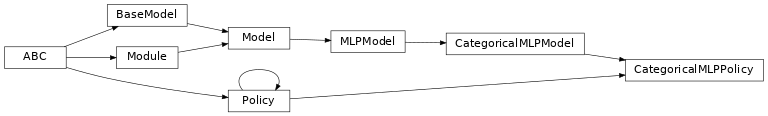Categorical MLP Policy.

A policy represented by a Categorical distribution which is parameterized by a multilayer perceptron (MLP).

It only works with akro.Discrete action space.

Parameters
• env_spec (garage.envs.env_spec.EnvSpec) – Environment specification.

• name (str) – Policy name, also the variable scope.

• hidden_sizes (list[int]) – Output dimension of dense layer(s). For example, (32, 32) means the MLP of this policy consists of two hidden layers, each with 32 hidden units.

• hidden_nonlinearity (callable) – Activation function for intermediate dense layer(s). It should return a tf.Tensor. Set it to None to maintain a linear activation.

• hidden_w_init (callable) – Initializer function for the weight of intermediate dense layer(s). The function should return a tf.Tensor.

• hidden_b_init (callable) – Initializer function for the bias of intermediate dense layer(s). The function should return a tf.Tensor.

• output_nonlinearity (callable) – Activation function for output dense layer. It should return a tf.Tensor. Set it to None to maintain a linear activation.

• output_w_init (callable) – Initializer function for the weight of output dense layer(s). The function should return a tf.Tensor.

• output_b_init (callable) – Initializer function for the bias of output dense layer(s). The function should return a tf.Tensor.

• layer_normalization (bool) – Bool for using layer normalization or not.

property input_dim(self)

int: Dimension of the policy input.

get_action(self, observation)

Return a single action.

Parameters

observation (numpy.ndarray) – Observations.

Returns

Action given input observation. dict(numpy.ndarray): Distribution parameters.

Return type

int

get_actions(self, observations)

Return multiple actions.

Parameters

observations (numpy.ndarray) – Observations.

Returns

Actions given input observations. dict(numpy.ndarray): Distribution parameters.

Return type

list[int]

get_regularizable_vars(self)

Get regularizable weight variables under the Policy scope.

Returns

Trainable variables.

Return type

list[tf.Tensor]

property env_spec(self)

Policy environment specification.

Returns

Environment specification.

Return type

garage.EnvSpec

clone(self, name)

Return a clone of the policy.

It copies the configuration of the primitive and also the parameters.

Parameters

name (str) – Name of the newly created policy. It has to be different from source policy if cloned under the same computational graph.

Returns

Newly cloned policy.

Return type

garage.tf.policies.Policy

network_output_spec(self)

Network output spec.

Returns

Name of the model outputs, in order.

Return type

list[str]

build(self, *inputs, name=None)

Build a Network with the given input(s).

* Do not call tf.global_variable_initializers() after building a model as it will reassign random weights to the model. The parameters inside a model will be initialized when calling build(). *

It uses the same, fixed variable scope for all Networks, to ensure parameter sharing. Different Networks must have an unique name.

Parameters
• inputs (list[tf.Tensor]) – Tensor input(s), recommended to be positional arguments, for example, def build(self, state_input, action_input, name=None).

• name (str) – Name of the model, which is also the name scope of the model.

Raises

ValueError – When a Network with the same name is already built.

Returns

Output tensors of the model with the given

inputs.

Return type

list[tf.Tensor]

network_input_spec(self)

Network input spec.

Returns

List of key(str) for the network inputs.

Return type

list[str]

property parameters(self)

Parameters of the model.

Returns

Parameters

Return type

np.ndarray

property name(self)

Name (str) of the model.

This is also the variable scope of the model.

Returns

Name of the model.

Return type

str

property input(self)

Default input of the model.

When the model is built the first time, by default it creates the ‘default’ network. This property creates a reference to the input of the network.

Returns

Default input of the model.

Return type

tf.Tensor

property output(self)

Default output of the model.

When the model is built the first time, by default it creates the ‘default’ network. This property creates a reference to the output of the network.

Returns

Default output of the model.

Return type

tf.Tensor

property inputs(self)

Default inputs of the model.

When the model is built the first time, by default it creates the ‘default’ network. This property creates a reference to the inputs of the network.

Returns

Default inputs of the model.

Return type

list[tf.Tensor]

property outputs(self)

Default outputs of the model.

When the model is built the first time, by default it creates the ‘default’ network. This property creates a reference to the outputs of the network.

Returns

Default outputs of the model.

Return type

list[tf.Tensor]

reset(self, do_resets=None)

Reset the module.

This is effective only to recurrent modules. do_resets is effective only to vectoried modules.

For a vectorized modules, do_resets is an array of boolean indicating which internal states to be reset. The length of do_resets should be equal to the length of inputs.

Parameters

do_resets (numpy.ndarray) – Bool array indicating which states to be reset.

property state_info_specs(self)

State info specification.

Returns

keys and shapes for the information related to the

module’s state when taking an action.

Return type

List[str]

property state_info_keys(self)

State info keys.

Returns

keys for the information related to the module’s state

when taking an input.

Return type

List[str]

terminate(self)

Clean up operation.

get_trainable_vars(self)

Get trainable variables.

Returns

A list of trainable variables in the current

variable scope.

Return type

List[tf.Variable]

get_global_vars(self)

Get global variables.

Returns

A list of global variables in the current

variable scope.

Return type

List[tf.Variable]

get_params(self)

Get the trainable variables.

Returns

A list of trainable variables in the current

variable scope.

Return type

List[tf.Variable]

get_param_shapes(self)

Get parameter shapes.

Returns

A list of variable shapes.

Return type

List[tuple]

get_param_values(self)

Get param values.

Returns

Values of the parameters evaluated in

the current session

Return type

np.ndarray

set_param_values(self, param_values)

Set param values.

Parameters

param_values (np.ndarray) – A numpy array of parameter values.

flat_to_params(self, flattened_params)

Unflatten tensors according to their respective shapes.

Parameters

flattened_params (np.ndarray) – A numpy array of flattened params.

Returns

A list of parameters reshaped to the

shapes specified.

Return type

List[np.ndarray]

property observation_space(self)

Observation space.

Returns

The observation space of the environment.

Return type

akro.Space

property action_space(self)

Action space.

Returns

The action space of the environment.

Return type

akro.Space

class ContinuousMLPPolicy(env_spec, name='ContinuousMLPPolicy', hidden_sizes=(64, 64), hidden_nonlinearity=tf.nn.relu, hidden_w_init=tf.initializers.glorot_uniform(seed=deterministic.get_tf_seed_stream()), hidden_b_init=tf.zeros_initializer(), output_nonlinearity=tf.nn.tanh, output_w_init=tf.initializers.glorot_uniform(seed=deterministic.get_tf_seed_stream()), output_b_init=tf.zeros_initializer(), layer_normalization=False)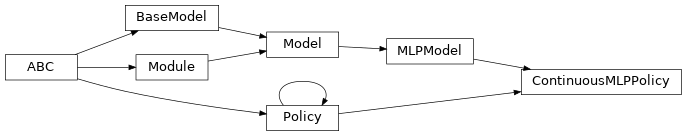Continuous MLP Policy Network.

The policy network selects action based on the state of the environment. It uses neural nets to fit the function of pi(s).

Parameters
• env_spec (garage.envs.env_spec.EnvSpec) – Environment specification.

• name (str) – Policy name, also the variable scope.

• hidden_sizes (list[int]) – Output dimension of dense layer(s). For example, (32, 32) means the MLP of this policy consists of two hidden layers, each with 32 hidden units.

• hidden_nonlinearity (callable) – Activation function for intermediate dense layer(s). It should return a tf.Tensor. Set it to None to maintain a linear activation.

• hidden_w_init (callable) – Initializer function for the weight of intermediate dense layer(s). The function should return a tf.Tensor.

• hidden_b_init (callable) – Initializer function for the bias of intermediate dense layer(s). The function should return a tf.Tensor.

• output_nonlinearity (callable) – Activation function for output dense layer. It should return a tf.Tensor. Set it to None to maintain a linear activation.

• output_w_init (callable) – Initializer function for the weight of output dense layer(s). The function should return a tf.Tensor.

• output_b_init (callable) – Initializer function for the bias of output dense layer(s). The function should return a tf.Tensor.

• layer_normalization (bool) – Bool for using layer normalization or not.

build(self, obs_var, name=None)

Symbolic graph of the action.

Parameters
• obs_var (tf.Tensor) – Tensor input for symbolic graph.

• name (str) – Name for symbolic graph.

Returns

symbolic graph of the action.

Return type

tf.Tensor

property input_dim(self)

int: Dimension of the policy input.

get_action(self, observation)

Get single action from this policy for the input observation.

Parameters

observation (numpy.ndarray) – Observation from environment.

Returns

Predicted action. dict: Empty dict since this policy does not model a distribution.

Return type

numpy.ndarray

get_actions(self, observations)

Get multiple actions from this policy for the input observations.

Parameters

observations (numpy.ndarray) – Observations from environment.

Returns

Predicted actions. dict: Empty dict since this policy does not model a distribution.

Return type

numpy.ndarray

get_regularizable_vars(self)

Get regularizable weight variables under the Policy scope.

Returns

List of regularizable variables.

Return type

list(tf.Variable)

property env_spec(self)

Policy environment specification.

Returns

Environment specification.

Return type

garage.EnvSpec

clone(self, name)

Return a clone of the policy.

It copies the configuration of the primitive and also the parameters.

Parameters

name (str) – Name of the newly created policy.

Returns

Clone of this object

Return type

garage.tf.policies.ContinuousMLPPolicy

network_input_spec(self)

Network input spec.

Returns

List of key(str) for the network inputs.

Return type

list[str]

network_output_spec(self)

Network output spec.

Returns

List of key(str) for the network outputs.

Return type

list[str]

property parameters(self)

Parameters of the model.

Returns

Parameters

Return type

np.ndarray

property name(self)

Name (str) of the model.

This is also the variable scope of the model.

Returns

Name of the model.

Return type

str

property input(self)

Default input of the model.

When the model is built the first time, by default it creates the ‘default’ network. This property creates a reference to the input of the network.

Returns

Default input of the model.

Return type

tf.Tensor

property output(self)

Default output of the model.

When the model is built the first time, by default it creates the ‘default’ network. This property creates a reference to the output of the network.

Returns

Default output of the model.

Return type

tf.Tensor

property inputs(self)

Default inputs of the model.

When the model is built the first time, by default it creates the ‘default’ network. This property creates a reference to the inputs of the network.

Returns

Default inputs of the model.

Return type

list[tf.Tensor]

property outputs(self)

Default outputs of the model.

When the model is built the first time, by default it creates the ‘default’ network. This property creates a reference to the outputs of the network.

Returns

Default outputs of the model.

Return type

list[tf.Tensor]

reset(self, do_resets=None)

Reset the module.

This is effective only to recurrent modules. do_resets is effective only to vectoried modules.

For a vectorized modules, do_resets is an array of boolean indicating which internal states to be reset. The length of do_resets should be equal to the length of inputs.

Parameters

do_resets (numpy.ndarray) – Bool array indicating which states to be reset.

property state_info_specs(self)

State info specification.

Returns

keys and shapes for the information related to the

module’s state when taking an action.

Return type

List[str]

property state_info_keys(self)

State info keys.

Returns

keys for the information related to the module’s state

when taking an input.

Return type

List[str]

terminate(self)

Clean up operation.

get_trainable_vars(self)

Get trainable variables.

Returns

A list of trainable variables in the current

variable scope.

Return type

List[tf.Variable]

get_global_vars(self)

Get global variables.

Returns

A list of global variables in the current

variable scope.

Return type

List[tf.Variable]

get_params(self)

Get the trainable variables.

Returns

A list of trainable variables in the current

variable scope.

Return type

List[tf.Variable]

get_param_shapes(self)

Get parameter shapes.

Returns

A list of variable shapes.

Return type

List[tuple]

get_param_values(self)

Get param values.

Returns

Values of the parameters evaluated in

the current session

Return type

np.ndarray

set_param_values(self, param_values)

Set param values.

Parameters

param_values (np.ndarray) – A numpy array of parameter values.

flat_to_params(self, flattened_params)

Unflatten tensors according to their respective shapes.

Parameters

flattened_params (np.ndarray) – A numpy array of flattened params.

Returns

A list of parameters reshaped to the

shapes specified.

Return type

List[np.ndarray]

property observation_space(self)

Observation space.

Returns

The observation space of the environment.

Return type

akro.Space

property action_space(self)

Action space.

Returns

The action space of the environment.

Return type

akro.Space

class DiscreteQFArgmaxPolicy(env_spec, qf, name='DiscreteQFArgmaxPolicy')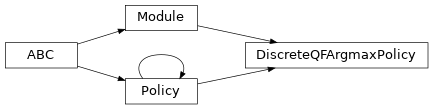DiscreteQFArgmax policy.

Parameters
• env_spec (garage.envs.env_spec.EnvSpec) – Environment specification.

• qf (garage.q_functions.QFunction) – The q-function used.

• name (str) – Name of the policy.

get_action(self, observation)

Get action from this policy for the input observation.

Parameters

observation (numpy.ndarray) – Observation from environment.

Returns

Single optimal action from this policy. dict: Predicted action and agent information. It returns an empty

dict since there is no parameterization.

Return type

numpy.ndarray

get_actions(self, observations)

Get actions from this policy for the input observations.

Parameters

observations (numpy.ndarray) – Observations from environment.

Returns

Optimal actions from this policy. dict: Predicted action and agent information. It returns an empty

dict since there is no parameterization.

Return type

numpy.ndarray

get_trainable_vars(self)

Get trainable variables.

Returns

A list of trainable variables in the current

variable scope.

Return type

List[tf.Variable]

get_global_vars(self)

Get global variables.

Returns

A list of global variables in the current

variable scope.

Return type

List[tf.Variable]

get_regularizable_vars(self)

Get all network weight variables in the current scope.

Returns

A list of network weight variables in the

current variable scope.

Return type

List[tf.Variable]

get_params(self)

Get the trainable variables.

Returns

A list of trainable variables in the current

variable scope.

Return type

List[tf.Variable]

get_param_shapes(self)

Get parameter shapes.

Returns

A list of variable shapes.

Return type

List[tuple]

get_param_values(self)

Get param values.

Returns

Values of the parameters evaluated in

the current session

Return type

np.ndarray

set_param_values(self, param_values)

Set param values.

Parameters

param_values (np.ndarray) – A numpy array of parameter values.

property env_spec(self)

Policy environment specification.

Returns

Environment specification.

Return type

garage.EnvSpec

property name(self)

str: Name of this module.

reset(self, do_resets=None)

Reset the module.

This is effective only to recurrent modules. do_resets is effective only to vectoried modules.

For a vectorized modules, do_resets is an array of boolean indicating which internal states to be reset. The length of do_resets should be equal to the length of inputs.

Parameters

do_resets (numpy.ndarray) – Bool array indicating which states to be reset.

property state_info_specs(self)

State info specification.

Returns

keys and shapes for the information related to the

module’s state when taking an action.

Return type

List[str]

property state_info_keys(self)

State info keys.

Returns

keys for the information related to the module’s state

when taking an input.

Return type

List[str]

terminate(self)

Clean up operation.

flat_to_params(self, flattened_params)

Unflatten tensors according to their respective shapes.

Parameters

flattened_params (np.ndarray) – A numpy array of flattened params.

Returns

A list of parameters reshaped to the

shapes specified.

Return type

List[np.ndarray]

property observation_space(self)

Observation space.

Returns

The observation space of the environment.

Return type

akro.Space

property action_space(self)

Action space.

Returns

The action space of the environment.

Return type

akro.Space

class GaussianGRUPolicy(env_spec, hidden_dim=32, name='GaussianGRUPolicy', hidden_nonlinearity=tf.nn.tanh, hidden_w_init=tf.initializers.glorot_uniform(seed=deterministic.get_tf_seed_stream()), hidden_b_init=tf.zeros_initializer(), recurrent_nonlinearity=tf.nn.sigmoid, recurrent_w_init=tf.initializers.glorot_uniform(seed=deterministic.get_tf_seed_stream()), output_nonlinearity=None, output_w_init=tf.initializers.glorot_uniform(seed=deterministic.get_tf_seed_stream()), output_b_init=tf.zeros_initializer(), hidden_state_init=tf.zeros_initializer(), hidden_state_init_trainable=False, learn_std=True, std_share_network=False, init_std=1.0, layer_normalization=False, state_include_action=True)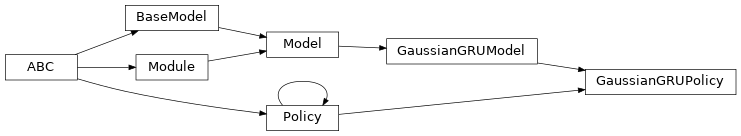Gaussian GRU Policy.

A policy represented by a Gaussian distribution which is parameterized by a Gated Recurrent Unit (GRU).

Parameters
• env_spec (garage.envs.env_spec.EnvSpec) – Environment specification.

• name (str) – Model name, also the variable scope.

• hidden_dim (int) – Hidden dimension for GRU cell for mean.

• hidden_nonlinearity (Callable) – Activation function for intermediate dense layer(s). It should return a tf.Tensor. Set it to None to maintain a linear activation.

• hidden_w_init (Callable) – Initializer function for the weight of intermediate dense layer(s). The function should return a tf.Tensor.

• hidden_b_init (Callable) – Initializer function for the bias of intermediate dense layer(s). The function should return a tf.Tensor.

• recurrent_nonlinearity (Callable) – Activation function for recurrent layers. It should return a tf.Tensor. Set it to None to maintain a linear activation.

• recurrent_w_init (Callable) – Initializer function for the weight of recurrent layer(s). The function should return a tf.Tensor.

• output_nonlinearity (Callable) – Activation function for output dense layer. It should return a tf.Tensor. Set it to None to maintain a linear activation.

• output_w_init (Callable) – Initializer function for the weight of output dense layer(s). The function should return a tf.Tensor.

• output_b_init (Callable) – Initializer function for the bias of output dense layer(s). The function should return a tf.Tensor.

• hidden_state_init (Callable) – Initializer function for the initial hidden state. The functino should return a tf.Tensor.

• hidden_state_init_trainable (bool) – Bool for whether the initial hidden state is trainable.

• learn_std (bool) – Is std trainable.

• std_share_network (bool) – Boolean for whether mean and std share the same network.

• init_std (float) – Initial value for std.

• layer_normalization (bool) – Bool for using layer normalization or not.

• state_include_action (bool) – Whether the state includes action. If True, input dimension will be (observation dimension + action dimension).

build(self, state_input, name=None)

Build policy.

Parameters
• state_input (tf.Tensor) – State input.

• name (str) – Name of the policy, which is also the name scope.

Returns

Policy distribution. tf.Tensor: Step means, with shape $$(N, S^*)$$. tf.Tensor: Step log std, with shape $$(N, S^*)$$. tf.Tensor: Step hidden state, with shape $$(N, S^*)$$. tf.Tensor: Initial hidden state, with shape $$(S^*)$$.

Return type

tfp.distributions.MultivariateNormalDiag

property input_dim(self)

int: Dimension of the policy input.

reset(self, do_resets=None)

Reset the policy.

Note

If do_resets is None, it will be by default np.array([True]) which implies the policy will not be “vectorized”, i.e. number of parallel environments for training data sampling = 1.

Parameters

do_resets (numpy.ndarray) – Bool that indicates terminal state(s).

get_action(self, observation)

Get single action from this policy for the input observation.

Parameters

observation (numpy.ndarray) – Observation from environment.

Returns

Actions dict: Predicted action and agent information.

Return type

numpy.ndarray

Note

It returns an action and a dict, with keys - mean (numpy.ndarray): Mean of the distribution. - log_std (numpy.ndarray): Log standard deviation of the

distribution.

• prev_action (numpy.ndarray): Previous action, only present if

self._state_include_action is True.

get_actions(self, observations)

Get multiple actions from this policy for the input observations.

Parameters

observations (numpy.ndarray) – Observations from environment.

Returns

Actions dict: Predicted action and agent information.

Return type

numpy.ndarray

Note

It returns an action and a dict, with keys - mean (numpy.ndarray): Means of the distribution. - log_std (numpy.ndarray): Log standard deviations of the

distribution.

• prev_action (numpy.ndarray): Previous action, only present if

self._state_include_action is True.

property state_info_specs(self)

State info specifcation.

Returns

keys and shapes for the information related to the

policy’s state when taking an action.

Return type

List[str]

property env_spec(self)

Policy environment specification.

Returns

Environment specification.

Return type

garage.EnvSpec

clone(self, name)

Return a clone of the policy.

It copies the configuration of the primitive and also the parameters.

Parameters

name (str) – Name of the newly created policy. It has to be different from source policy if cloned under the same computational graph.

Returns

Newly cloned policy.

Return type

garage.tf.policies.GaussianGRUPolicy

network_input_spec(self)

Network input spec.

Returns

Name of the model inputs, in order.

Return type

list[str]

network_output_spec(self)

Network output spec.

Returns

Name of the model outputs, in order.

Return type

list[str]

property parameters(self)

Parameters of the model.

Returns

Parameters

Return type

np.ndarray

property name(self)

Name (str) of the model.

This is also the variable scope of the model.

Returns

Name of the model.

Return type

str

property input(self)

Default input of the model.

When the model is built the first time, by default it creates the ‘default’ network. This property creates a reference to the input of the network.

Returns

Default input of the model.

Return type

tf.Tensor

property output(self)

Default output of the model.

When the model is built the first time, by default it creates the ‘default’ network. This property creates a reference to the output of the network.

Returns

Default output of the model.

Return type

tf.Tensor

property inputs(self)

Default inputs of the model.

When the model is built the first time, by default it creates the ‘default’ network. This property creates a reference to the inputs of the network.

Returns

Default inputs of the model.

Return type

list[tf.Tensor]

property outputs(self)

Default outputs of the model.

When the model is built the first time, by default it creates the ‘default’ network. This property creates a reference to the outputs of the network.

Returns

Default outputs of the model.

Return type

list[tf.Tensor]

property state_info_keys(self)

State info keys.

Returns

keys for the information related to the module’s state

when taking an input.

Return type

List[str]

terminate(self)

Clean up operation.

get_trainable_vars(self)

Get trainable variables.

Returns

A list of trainable variables in the current

variable scope.

Return type

List[tf.Variable]

get_global_vars(self)

Get global variables.

Returns

A list of global variables in the current

variable scope.

Return type

List[tf.Variable]

get_regularizable_vars(self)

Get all network weight variables in the current scope.

Returns

A list of network weight variables in the

current variable scope.

Return type

List[tf.Variable]

get_params(self)

Get the trainable variables.

Returns

A list of trainable variables in the current

variable scope.

Return type

List[tf.Variable]

get_param_shapes(self)

Get parameter shapes.

Returns

A list of variable shapes.

Return type

List[tuple]

get_param_values(self)

Get param values.

Returns

Values of the parameters evaluated in

the current session

Return type

np.ndarray

set_param_values(self, param_values)

Set param values.

Parameters

param_values (np.ndarray) – A numpy array of parameter values.

flat_to_params(self, flattened_params)

Unflatten tensors according to their respective shapes.

Parameters

flattened_params (np.ndarray) – A numpy array of flattened params.

Returns

A list of parameters reshaped to the

shapes specified.

Return type

List[np.ndarray]

property observation_space(self)

Observation space.

Returns

The observation space of the environment.

Return type

akro.Space

property action_space(self)

Action space.

Returns

The action space of the environment.

Return type

akro.Space

class GaussianLSTMPolicy(env_spec, hidden_dim=32, name='GaussianLSTMPolicy', hidden_nonlinearity=tf.nn.tanh, hidden_w_init=tf.initializers.glorot_uniform(seed=deterministic.get_tf_seed_stream()), hidden_b_init=tf.zeros_initializer(), recurrent_nonlinearity=tf.nn.sigmoid, recurrent_w_init=tf.initializers.glorot_uniform(seed=deterministic.get_tf_seed_stream()), output_nonlinearity=None, output_w_init=tf.initializers.glorot_uniform(seed=deterministic.get_tf_seed_stream()), output_b_init=tf.zeros_initializer(), hidden_state_init=tf.zeros_initializer(), hidden_state_init_trainable=False, cell_state_init=tf.zeros_initializer(), cell_state_init_trainable=False, forget_bias=True, learn_std=True, std_share_network=False, init_std=1.0, layer_normalization=False, state_include_action=True)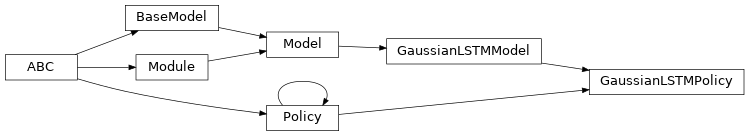Gaussian LSTM Policy.

A policy represented by a Gaussian distribution which is parameterized by a Long short-term memory (LSTM).

Parameters
• env_spec (garage.envs.env_spec.EnvSpec) – Environment specification.

• name (str) – Model name, also the variable scope.

• hidden_dim (int) – Hidden dimension for LSTM cell for mean.

• hidden_nonlinearity (Callable) – Activation function for intermediate dense layer(s). It should return a tf.Tensor. Set it to None to maintain a linear activation.

• hidden_w_init (Callable) – Initializer function for the weight of intermediate dense layer(s). The function should return a tf.Tensor.

• hidden_b_init (Callable) – Initializer function for the bias of intermediate dense layer(s). The function should return a tf.Tensor.

• recurrent_nonlinearity (Callable) – Activation function for recurrent layers. It should return a tf.Tensor. Set it to None to maintain a linear activation.

• recurrent_w_init (Callable) – Initializer function for the weight of recurrent layer(s). The function should return a tf.Tensor.

• output_nonlinearity (Callable) – Activation function for output dense layer. It should return a tf.Tensor. Set it to None to maintain a linear activation.

• output_w_init (Callable) – Initializer function for the weight of output dense layer(s). The function should return a tf.Tensor.

• output_b_init (Callable) – Initializer function for the bias of output dense layer(s). The function should return a tf.Tensor.

• hidden_state_init (Callable) – Initializer function for the initial hidden state. The functino should return a tf.Tensor.

• hidden_state_init_trainable (bool) – Bool for whether the initial hidden state is trainable.

• cell_state_init (Callable) – Initializer function for the initial cell state. The functino should return a tf.Tensor.

• cell_state_init_trainable (bool) – Bool for whether the initial cell state is trainable.

• forget_bias (bool) – If True, add 1 to the bias of the forget gate at initialization. It’s used to reduce the scale of forgetting at the beginning of the training.

• learn_std (bool) – Is std trainable.

• std_share_network (bool) – Boolean for whether mean and std share the same network.

• init_std (float) – Initial value for std.

• layer_normalization (bool) – Bool for using layer normalization or not.

• state_include_action (bool) – Whether the state includes action. If True, input dimension will be (observation dimension + action dimension).

build(self, state_input, name=None)

Build policy.

Parameters
• state_input (tf.Tensor) – State input.

• name (str) – Name of the policy, which is also the name scope.

Returns

Policy distribution. tf.Tensor: Step means, with shape $$(N, S^*)$$. tf.Tensor: Step log std, with shape $$(N, S^*)$$. tf.Tensor: Step hidden state, with shape $$(N, S^*)$$. tf.Tensor: Step cell state, with shape $$(N, S^*)$$. tf.Tensor: Initial hidden state, with shape $$(S^*)$$. tf.Tensor: Initial cell state, with shape $$(S^*)$$

Return type

tfp.distributions.MultivariateNormalDiag

property input_dim(self)

int: Dimension of the policy input.

reset(self, do_resets=None)

Reset the policy.

Note

If do_resets is None, it will be by default np.array([True]), which implies the policy will not be “vectorized”, i.e. number of paralle environments for training data sampling = 1.

Parameters

do_resets (numpy.ndarray) – Bool that indicates terminal state(s).

get_action(self, observation)

Get single action from this policy for the input observation.

Parameters

observation (numpy.ndarray) – Observation from environment.

Returns

Actions dict: Predicted action and agent information.

Return type

numpy.ndarray

Note

It returns an action and a dict, with keys - mean (numpy.ndarray): Mean of the distribution. - log_std (numpy.ndarray): Log standard deviation of the

distribution.

• prev_action (numpy.ndarray): Previous action, only present if

self._state_include_action is True.

get_actions(self, observations)

Get multiple actions from this policy for the input observations.

Parameters

observations (numpy.ndarray) – Observations from environment.

Returns

Actions dict: Predicted action and agent information.

Return type

numpy.ndarray

Note

It returns an action and a dict, with keys - mean (numpy.ndarray): Means of the distribution. - log_std (numpy.ndarray): Log standard deviations of the

distribution.

• prev_action (numpy.ndarray): Previous action, only present if

self._state_include_action is True.

property state_info_specs(self)

State info specifcation.

Returns

keys and shapes for the information related to the

policy’s state when taking an action.

Return type

List[str]

property env_spec(self)

Policy environment specification.

Returns

Environment specification.

Return type

garage.EnvSpec

clone(self, name)

Return a clone of the policy.

It copies the configuration of the primitive and also the parameters.

Parameters

name (str) – Name of the newly created policy. It has to be different from source policy if cloned under the same computational graph.

Returns

Newly cloned policy.

Return type

garage.tf.policies.GaussianLSTMPolicy

network_input_spec(self)

Network input spec.

Returns

Name of the model inputs, in order.

Return type

list[str]

network_output_spec(self)

Network output spec.

Returns

Name of the model outputs, in order.

Return type

list[str]

property parameters(self)

Parameters of the model.

Returns

Parameters

Return type

np.ndarray

property name(self)

Name (str) of the model.

This is also the variable scope of the model.

Returns

Name of the model.

Return type

str

property input(self)

Default input of the model.

When the model is built the first time, by default it creates the ‘default’ network. This property creates a reference to the input of the network.

Returns

Default input of the model.

Return type

tf.Tensor

property output(self)

Default output of the model.

When the model is built the first time, by default it creates the ‘default’ network. This property creates a reference to the output of the network.

Returns

Default output of the model.

Return type

tf.Tensor

property inputs(self)

Default inputs of the model.

When the model is built the first time, by default it creates the ‘default’ network. This property creates a reference to the inputs of the network.

Returns

Default inputs of the model.

Return type

list[tf.Tensor]

property outputs(self)

Default outputs of the model.

When the model is built the first time, by default it creates the ‘default’ network. This property creates a reference to the outputs of the network.

Returns

Default outputs of the model.

Return type

list[tf.Tensor]

property state_info_keys(self)

State info keys.

Returns

keys for the information related to the module’s state

when taking an input.

Return type

List[str]

terminate(self)

Clean up operation.

get_trainable_vars(self)

Get trainable variables.

Returns

A list of trainable variables in the current

variable scope.

Return type

List[tf.Variable]

get_global_vars(self)

Get global variables.

Returns

A list of global variables in the current

variable scope.

Return type

List[tf.Variable]

get_regularizable_vars(self)

Get all network weight variables in the current scope.

Returns

A list of network weight variables in the

current variable scope.

Return type

List[tf.Variable]

get_params(self)

Get the trainable variables.

Returns

A list of trainable variables in the current

variable scope.

Return type

List[tf.Variable]

get_param_shapes(self)

Get parameter shapes.

Returns

A list of variable shapes.

Return type

List[tuple]

get_param_values(self)

Get param values.

Returns

Values of the parameters evaluated in

the current session

Return type

np.ndarray

set_param_values(self, param_values)

Set param values.

Parameters

param_values (np.ndarray) – A numpy array of parameter values.

flat_to_params(self, flattened_params)

Unflatten tensors according to their respective shapes.

Parameters

flattened_params (np.ndarray) – A numpy array of flattened params.

Returns

A list of parameters reshaped to the

shapes specified.

Return type

List[np.ndarray]

property observation_space(self)

Observation space.

Returns

The observation space of the environment.

Return type

akro.Space

property action_space(self)

Action space.

Returns

The action space of the environment.

Return type

akro.Space

class GaussianMLPPolicy(env_spec, name='GaussianMLPPolicy', hidden_sizes=(32, 32), hidden_nonlinearity=tf.nn.tanh, hidden_w_init=tf.initializers.glorot_uniform(seed=deterministic.get_tf_seed_stream()), hidden_b_init=tf.zeros_initializer(), output_nonlinearity=None, output_w_init=tf.initializers.glorot_uniform(seed=deterministic.get_tf_seed_stream()), output_b_init=tf.zeros_initializer(), learn_std=True, adaptive_std=False, std_share_network=False, init_std=1.0, min_std=1e-06, max_std=None, std_hidden_sizes=(32, 32), std_hidden_nonlinearity=tf.nn.tanh, std_output_nonlinearity=None, std_parameterization='exp', layer_normalization=False)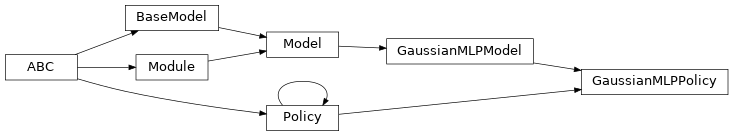Gaussian MLP Policy.

A policy represented by a Gaussian distribution which is parameterized by a multilayer perceptron (MLP).

Parameters
• env_spec (garage.envs.env_spec.EnvSpec) – Environment specification.

• name (str) – Model name, also the variable scope.

• hidden_sizes (list[int]) – Output dimension of dense layer(s) for the MLP for mean. For example, (32, 32) means the MLP consists of two hidden layers, each with 32 hidden units.

• hidden_nonlinearity (callable) – Activation function for intermediate dense layer(s). It should return a tf.Tensor. Set it to None to maintain a linear activation.

• hidden_w_init (callable) – Initializer function for the weight of intermediate dense layer(s). The function should return a tf.Tensor.

• hidden_b_init (callable) – Initializer function for the bias of intermediate dense layer(s). The function should return a tf.Tensor.

• output_nonlinearity (callable) – Activation function for output dense layer. It should return a tf.Tensor. Set it to None to maintain a linear activation.

• output_w_init (callable) – Initializer function for the weight of output dense layer(s). The function should return a tf.Tensor.

• output_b_init (callable) – Initializer function for the bias of output dense layer(s). The function should return a tf.Tensor.

• learn_std (bool) – Is std trainable.

• adaptive_std (bool) – Is std a neural network. If False, it will be a parameter.

• std_share_network (bool) – Boolean for whether mean and std share the same network.

• init_std (float) – Initial value for std.

• std_hidden_sizes (list[int]) – Output dimension of dense layer(s) for the MLP for std. For example, (32, 32) means the MLP consists of two hidden layers, each with 32 hidden units.

• min_std (float) – If not None, the std is at least the value of min_std, to avoid numerical issues.

• max_std (float) – If not None, the std is at most the value of max_std, to avoid numerical issues.

• std_hidden_nonlinearity (callable) – Nonlinearity for each hidden layer in the std network. The function should return a tf.Tensor.

• std_output_nonlinearity (callable) – Nonlinearity for output layer in the std network. The function should return a tf.Tensor.

• std_parameterization (str) – How the std should be parametrized. There are a few options:

• exp (-) – the logarithm of the std will be stored, and applied a exponential transformation

• softplus (-) – the std will be computed as log(1+exp(x))

• layer_normalization (bool) – Bool for using layer normalization or not.

property input_dim(self)

int: Dimension of the policy input.

get_action(self, observation)

Get single action from this policy for the input observation.

Parameters

observation (numpy.ndarray) – Observation from environment.

Returns

Actions dict: Predicted action and agent information.

Return type

numpy.ndarray

Note

It returns an action and a dict, with keys - mean (numpy.ndarray): Mean of the distribution. - log_std (numpy.ndarray): Log standard deviation of the

distribution.

get_actions(self, observations)

Get multiple actions from this policy for the input observations.

Parameters

observations (numpy.ndarray) – Observations from environment.

Returns

Actions dict: Predicted action and agent information.

Return type

numpy.ndarray

Note

It returns actions and a dict, with keys - mean (numpy.ndarray): Means of the distribution. - log_std (numpy.ndarray): Log standard deviations of the

distribution.

clone(self, name)

Return a clone of the policy.

It copies the configuration of the primitive and also the parameters.

Parameters

name (str) – Name of the newly created policy. It has to be different from source policy if cloned under the same computational graph.

Returns

Newly cloned policy.

Return type

garage.tf.policies.GaussianMLPPolicy

property env_spec(self)

Policy environment specification.

Returns

Environment specification.

Return type

garage.EnvSpec

network_output_spec(self)

Network output spec.

Returns

List of key(str) for the network outputs.

Return type

list[str]

build(self, *inputs, name=None)

Build a Network with the given input(s).

* Do not call tf.global_variable_initializers() after building a model as it will reassign random weights to the model. The parameters inside a model will be initialized when calling build(). *

It uses the same, fixed variable scope for all Networks, to ensure parameter sharing. Different Networks must have an unique name.

Parameters
• inputs (list[tf.Tensor]) – Tensor input(s), recommended to be positional arguments, for example, def build(self, state_input, action_input, name=None).

• name (str) – Name of the model, which is also the name scope of the model.

Raises

ValueError – When a Network with the same name is already built.

Returns

Output tensors of the model with the given

inputs.

Return type

list[tf.Tensor]

network_input_spec(self)

Network input spec.

Returns

List of key(str) for the network inputs.

Return type

list[str]

property parameters(self)

Parameters of the model.

Returns

Parameters

Return type

np.ndarray

property name(self)

Name (str) of the model.

This is also the variable scope of the model.

Returns

Name of the model.

Return type

str

property input(self)

Default input of the model.

When the model is built the first time, by default it creates the ‘default’ network. This property creates a reference to the input of the network.

Returns

Default input of the model.

Return type

tf.Tensor

property output(self)

Default output of the model.

When the model is built the first time, by default it creates the ‘default’ network. This property creates a reference to the output of the network.

Returns

Default output of the model.

Return type

tf.Tensor

property inputs(self)

Default inputs of the model.

When the model is built the first time, by default it creates the ‘default’ network. This property creates a reference to the inputs of the network.

Returns

Default inputs of the model.

Return type

list[tf.Tensor]

property outputs(self)

Default outputs of the model.

When the model is built the first time, by default it creates the ‘default’ network. This property creates a reference to the outputs of the network.

Returns

Default outputs of the model.

Return type

list[tf.Tensor]

reset(self, do_resets=None)

Reset the module.

This is effective only to recurrent modules. do_resets is effective only to vectoried modules.

For a vectorized modules, do_resets is an array of boolean indicating which internal states to be reset. The length of do_resets should be equal to the length of inputs.

Parameters

do_resets (numpy.ndarray) – Bool array indicating which states to be reset.

property state_info_specs(self)

State info specification.

Returns

keys and shapes for the information related to the

module’s state when taking an action.

Return type

List[str]

property state_info_keys(self)

State info keys.

Returns

keys for the information related to the module’s state

when taking an input.

Return type

List[str]

terminate(self)

Clean up operation.

get_trainable_vars(self)

Get trainable variables.

Returns

A list of trainable variables in the current

variable scope.

Return type

List[tf.Variable]

get_global_vars(self)

Get global variables.

Returns

A list of global variables in the current

variable scope.

Return type

List[tf.Variable]

get_regularizable_vars(self)

Get all network weight variables in the current scope.

Returns

A list of network weight variables in the

current variable scope.

Return type

List[tf.Variable]

get_params(self)

Get the trainable variables.

Returns

A list of trainable variables in the current

variable scope.

Return type

List[tf.Variable]

get_param_shapes(self)

Get parameter shapes.

Returns

A list of variable shapes.

Return type

List[tuple]

get_param_values(self)

Get param values.

Returns

Values of the parameters evaluated in

the current session

Return type

np.ndarray

set_param_values(self, param_values)

Set param values.

Parameters

param_values (np.ndarray) – A numpy array of parameter values.

flat_to_params(self, flattened_params)

Unflatten tensors according to their respective shapes.

Parameters

flattened_params (np.ndarray) – A numpy array of flattened params.

Returns

A list of parameters reshaped to the

shapes specified.

Return type

List[np.ndarray]

property observation_space(self)

Observation space.

Returns

The observation space of the environment.

Return type

akro.Space

property action_space(self)

Action space.

Returns

The action space of the environment.

Return type

akro.Space

class GaussianMLPTaskEmbeddingPolicy(env_spec, encoder, name='GaussianMLPTaskEmbeddingPolicy', hidden_sizes=(32, 32), hidden_nonlinearity=tf.nn.tanh, hidden_w_init=tf.initializers.glorot_uniform(seed=deterministic.get_tf_seed_stream()), hidden_b_init=tf.zeros_initializer(), output_nonlinearity=None, output_w_init=tf.initializers.glorot_uniform(seed=deterministic.get_tf_seed_stream()), output_b_init=tf.zeros_initializer(), learn_std=True, adaptive_std=False, std_share_network=False, init_std=1.0, min_std=1e-06, max_std=None, std_hidden_sizes=(32, 32), std_hidden_nonlinearity=tf.nn.tanh, std_output_nonlinearity=None, std_parameterization='exp', layer_normalization=False)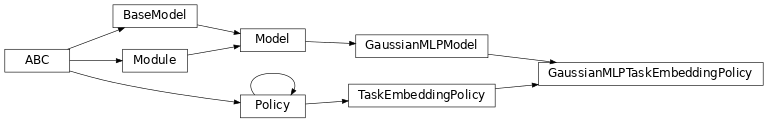Parameters
• env_spec (garage.envs.env_spec.EnvSpec) – Environment specification.

• encoder (garage.tf.embeddings.StochasticEncoder) – Embedding network.

• name (str) – Model name, also the variable scope.

• hidden_sizes (list[int]) – Output dimension of dense layer(s) for the MLP for mean. For example, (32, 32) means the MLP consists of two hidden layers, each with 32 hidden units.

• hidden_nonlinearity (callable) – Activation function for intermediate dense layer(s). It should return a tf.Tensor. Set it to None to maintain a linear activation.

• hidden_w_init (callable) – Initializer function for the weight of intermediate dense layer(s). The function should return a tf.Tensor.

• hidden_b_init (callable) – Initializer function for the bias of intermediate dense layer(s). The function should return a tf.Tensor.

• output_nonlinearity (callable) – Activation function for output dense layer. It should return a tf.Tensor. Set it to None to maintain a linear activation.

• output_w_init (callable) – Initializer function for the weight of output dense layer(s). The function should return a tf.Tensor.

• output_b_init (callable) – Initializer function for the bias of output dense layer(s). The function should return a tf.Tensor.

• learn_std (bool) – Is std trainable.

• adaptive_std (bool) – Is std a neural network. If False, it will be a parameter.

• std_share_network (bool) – Boolean for whether mean and std share the same network.

• init_std (float) – Initial value for std.

• std_hidden_sizes (list[int]) – Output dimension of dense layer(s) for the MLP for std. For example, (32, 32) means the MLP consists of two hidden layers, each with 32 hidden units.

• min_std (float) – If not None, the std is at least the value of min_std, to avoid numerical issues.

• max_std (float) – If not None, the std is at most the value of max_std, to avoid numerical issues.

• std_hidden_nonlinearity (callable) – Nonlinearity for each hidden layer in the std network. It should return a tf.Tensor. Set it to None to maintain a linear activation.

• std_output_nonlinearity (callable) – Nonlinearity for output layer in the std network. It should return a tf.Tensor. Set it to None to maintain a linear activation.

• std_parameterization (str) –

How the std should be parametrized. There are a few options: - exp: the logarithm of the std will be stored, and applied a

exponential transformation

• softplus: the std will be computed as log(1+exp(x))

• layer_normalization (bool) – Bool for using layer normalization or not.

build(self, obs_input, task_input, name=None)

Build policy.

Parameters
• obs_input (tf.Tensor) – Observation input.

• name (str) – Name of the model, which is also the name scope.

Returns

Policy network. namedtuple: Encoder network.

Return type

namedtuple

get_action(self, observation)

Get action sampled from the policy.

Parameters

observation (np.ndarray) – Augmented observation from the environment, with shape $$(O+N, )$$. O is the dimension of observation, N is the number of tasks.

Returns

Action sampled from the policy,

with shape $$(A, )$$. A is the dimension of action.

dict: Action distribution information, with keys:
• mean (numpy.ndarray): Mean of the distribution,

with shape $$(A, )$$. A is the dimension of action.

• log_std (numpy.ndarray): Log standard deviation of the

distribution, with shape $$(A, )$$. A is the dimension of action.

Return type

np.ndarray

get_actions(self, observations)

Get actions sampled from the policy.

Parameters

observations (np.ndarray) – Augmented observation from the environment, with shape $$(T, O+N)$$. T is the number of environment steps, O is the dimension of observation, N is the number of tasks.

Returns

Actions sampled from the policy,

with shape $$(T, A)$$. T is the number of environment steps, A is the dimension of action.

dict: Action distribution information, with keys:
• mean (numpy.ndarray): Mean of the distribution,

with shape $$(T, A)$$. T is the number of environment steps, A is the dimension of action.

• log_std (numpy.ndarray): Log standard deviation of the

distribution, with shape $$(T, A)$$. T is the number of environment steps, Z is the dimension of action.

Return type

np.ndarray

get_action_given_latent(self, observation, latent)

Sample an action given observation and latent.

Parameters
• observation (np.ndarray) – Observation from the environment, with shape $$(O, )$$. O is the dimension of observation.

• latent (np.ndarray) – Latent, with shape $$(Z, )$$. Z is the dimension of the latent embedding.

Returns

Action sampled from the policy,

with shape $$(A, )$$. A is the dimension of action.

dict: Action distribution information, with keys:
• mean (numpy.ndarray): Mean of the distribution,

with shape $$(A, )$$. A is the dimension of action.

• log_std (numpy.ndarray): Log standard deviation of the

distribution, with shape $$(A, )$$. A is the dimension of action.

Return type

np.ndarray

get_actions_given_latents(self, observations, latents)

Sample a batch of actions given observations and latents.

Parameters
• observations (np.ndarray) – Observations from the environment, with shape $$(T, O)$$. T is the number of environment steps, O is the dimension of observation.

• latents (np.ndarray) – Latents, with shape $$(T, Z)$$. T is the number of environment steps, Z is the dimension of latent embedding.

Returns

Actions sampled from the policy,

with shape $$(T, A)$$. T is the number of environment steps, A is the dimension of action.

dict: Action distribution information, , with keys:
• mean (numpy.ndarray): Mean of the distribution,

with shape $$(T, A)$$. T is the number of environment steps. A is the dimension of action.

• log_std (numpy.ndarray): Log standard deviation of the

distribution, with shape $$(T, A)$$. T is the number of environment steps. A is the dimension of action.

Return type

np.ndarray

get_action_given_task(self, observation, task_id)

Sample an action given observation and task id.

Parameters
• observation (np.ndarray) – Observation from the environment, with shape $$(O, )$$. O is the dimension of the observation.

• task_id (np.ndarray) – One-hot task id, with shape :math:(N, ). N is the number of tasks.

Returns

Action sampled from the policy, with shape

$$(A, )$$. A is the dimension of action.

dict: Action distribution information, with keys:
• mean (numpy.ndarray): Mean of the distribution,

with shape $$(A, )$$. A is the dimension of action.

• log_std (numpy.ndarray): Log standard deviation of the

distribution, with shape $$(A, )$$. A is the dimension of action.

Return type

np.ndarray

get_actions_given_tasks(self, observations, task_ids)

Sample a batch of actions given observations and task ids.

Parameters
• observations (np.ndarray) – Observations from the environment, with shape $$(T, O)$$. T is the number of environment steps, O is the dimension of observation.

• task_ids (np.ndarry) – One-hot task ids, with shape $$(T, N)$$. T is the number of environment steps, N is the number of tasks.

Returns

Actions sampled from the policy,

with shape $$(T, A)$$. T is the number of environment steps, A is the dimension of action.

dict: Action distribution information, , with keys:
• mean (numpy.ndarray): Mean of the distribution,

with shape $$(T, A)$$. T is the number of environment steps. A is the dimension of action.

• log_std (numpy.ndarray): Log standard deviation of the

distribution, with shape $$(T, A)$$. T is the number of environment steps. A is the dimension of action.

Return type

np.ndarray

get_trainable_vars(self)

Get trainable variables.

The trainable vars of a multitask policy should be the trainable vars of its model and the trainable vars of its embedding model.

Returns

A list of trainable variables in the current

variable scope.

Return type

List[tf.Variable]

get_global_vars(self)

Get global variables.

The global vars of a multitask policy should be the global vars of its model and the trainable vars of its embedding model.

Returns

A list of global variables in the current

variable scope.

Return type

List[tf.Variable]

property env_spec(self)

Policy environment specification.

Returns

Environment specification.

Return type

garage.EnvSpec

property encoder(self)

garage.tf.embeddings.encoder.Encoder: Encoder.

property augmented_observation_space(self)

akro.Box: Concatenated observation space and one-hot task id.

clone(self, name)

Return a clone of the policy.

It copies the configuration of the primitive and also the parameters.

Parameters

name (str) – Name of the newly created policy. It has to be different from source policy if cloned under the same computational graph.

Returns

Cloned policy.

Return type

network_output_spec(self)

Network output spec.

Returns

List of key(str) for the network outputs.

Return type

list[str]

network_input_spec(self)

Network input spec.

Returns

List of key(str) for the network inputs.

Return type

list[str]

property parameters(self)

Parameters of the model.

Returns

Parameters

Return type

np.ndarray

property name(self)

Name (str) of the model.

This is also the variable scope of the model.

Returns

Name of the model.

Return type

str

property input(self)

Default input of the model.

When the model is built the first time, by default it creates the ‘default’ network. This property creates a reference to the input of the network.

Returns

Default input of the model.

Return type

tf.Tensor

property output(self)

Default output of the model.

When the model is built the first time, by default it creates the ‘default’ network. This property creates a reference to the output of the network.

Returns

Default output of the model.

Return type

tf.Tensor

property inputs(self)

Default inputs of the model.

When the model is built the first time, by default it creates the ‘default’ network. This property creates a reference to the inputs of the network.

Returns

Default inputs of the model.

Return type

list[tf.Tensor]

property outputs(self)

Default outputs of the model.

When the model is built the first time, by default it creates the ‘default’ network. This property creates a reference to the outputs of the network.

Returns

Default outputs of the model.

Return type

list[tf.Tensor]

reset(self, do_resets=None)

Reset the module.

This is effective only to recurrent modules. do_resets is effective only to vectoried modules.

For a vectorized modules, do_resets is an array of boolean indicating which internal states to be reset. The length of do_resets should be equal to the length of inputs.

Parameters

do_resets (numpy.ndarray) – Bool array indicating which states to be reset.

property state_info_specs(self)

State info specification.

Returns

keys and shapes for the information related to the

module’s state when taking an action.

Return type

List[str]

property state_info_keys(self)

State info keys.

Returns

keys for the information related to the module’s state

when taking an input.

Return type

List[str]

terminate(self)

Clean up operation.

get_regularizable_vars(self)

Get all network weight variables in the current scope.

Returns

A list of network weight variables in the

current variable scope.

Return type

List[tf.Variable]

get_params(self)

Get the trainable variables.

Returns

A list of trainable variables in the current

variable scope.

Return type

List[tf.Variable]

get_param_shapes(self)

Get parameter shapes.

Returns

A list of variable shapes.

Return type

List[tuple]

get_param_values(self)

Get param values.

Returns

Values of the parameters evaluated in

the current session

Return type

np.ndarray

set_param_values(self, param_values)

Set param values.

Parameters

param_values (np.ndarray) – A numpy array of parameter values.

flat_to_params(self, flattened_params)

Unflatten tensors according to their respective shapes.

Parameters

flattened_params (np.ndarray) – A numpy array of flattened params.

Returns

A list of parameters reshaped to the

shapes specified.

Return type

List[np.ndarray]

get_latent(self, task_id)

Get embedded task id in latent space.

Parameters

task_id (np.ndarray) – One-hot task id, with shape $$(N, )$$. N is the number of tasks.

Returns

An embedding sampled from embedding distribution, with

shape $$(Z, )$$. Z is the dimension of the latent embedding.

dict: Embedding distribution information.

Return type

np.ndarray

property latent_space(self)

akro.Box: Space of latent.

property task_space(self)

akro.Box: One-hot space of task id.

property encoder_distribution(self)

tfp.Distribution.MultivariateNormalDiag: Encoder distribution.

split_augmented_observation(self, collated)

Splits up observation into one-hot task and environment observation.

Parameters

collated (np.ndarray) – Environment observation concatenated with task one-hot, with shape $$(O+N, )$$. O is the dimension of observation, N is the number of tasks.

Returns

Vanilla environment observation,

with shape $$(O, )$$. O is the dimension of observation.

np.ndarray: Task one-hot, with shape $$(N, )$$. N is the number

Return type

np.ndarray

property observation_space(self)

Observation space.

Returns

The observation space of the environment.

Return type

akro.Space

property action_space(self)

Action space.

Returns

The action space of the environment.

Return type

akro.Space

class Policy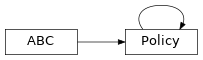Base class for policies in TensorFlow.

abstract get_action(self, observation)

Get action sampled from the policy.

Parameters

observation (np.ndarray) – Observation from the environment.

Returns

Action and extra agent

info.

Return type

Tuple[np.ndarray, dict[str,np.ndarray]]

abstract get_actions(self, observations)

Get actions given observations.

Parameters

observations (np.ndarray) – Observations from the environment.

Returns

Actions and extra agent

infos.

Return type

Tuple[np.ndarray, dict[str,np.ndarray]]

property state_info_specs(self)

State info specification.

Returns

keys and shapes for the information related to the

module’s state when taking an action.

Return type

List[str]

property state_info_keys(self)

State info keys.

Returns

keys for the information related to the module’s state

when taking an input.

Return type

List[str]

reset(self, do_resets=None)

Reset the policy.

This is effective only to recurrent policies.

do_resets is an array of boolean indicating which internal states to be reset. The length of do_resets should be equal to the length of inputs, i.e. batch size.

Parameters

do_resets (numpy.ndarray) – Bool array indicating which states to be reset.

property name(self)

Name of policy.

Returns

Name of policy

Return type

str

property env_spec(self)

Policy environment specification.

Returns

Environment specification.

Return type

garage.EnvSpec

property observation_space(self)

Observation space.

Returns

The observation space of the environment.

Return type

akro.Space

property action_space(self)

Action space.

Returns

The action space of the environment.

Return type

akro.Space

class TaskEmbeddingPolicy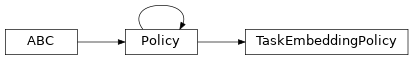Base class for Task Embedding policies in TensorFlow.

This policy needs a task id in addition to observation to sample an action.

property encoder(self)

garage.tf.embeddings.encoder.Encoder: Encoder.

get_latent(self, task_id)

Get embedded task id in latent space.

Parameters

task_id (np.ndarray) – One-hot task id, with shape $$(N, )$$. N is the number of tasks.

Returns

An embedding sampled from embedding distribution, with

shape $$(Z, )$$. Z is the dimension of the latent embedding.

dict: Embedding distribution information.

Return type

np.ndarray

property latent_space(self)

akro.Box: Space of latent.

property task_space(self)

akro.Box: One-hot space of task id.

property augmented_observation_space(self)

akro.Box: Concatenated observation space and one-hot task id.

property encoder_distribution(self)

tfp.Distribution.MultivariateNormalDiag: Encoder distribution.

abstract get_action(self, observation)

Get action sampled from the policy.

Parameters

observation (np.ndarray) – Augmented observation from the environment, with shape $$(O+N, )$$. O is the dimension of observation, N is the number of tasks.

Returns

Action sampled from the policy,

with shape $$(A, )$$. A is the dimension of action.

dict: Action distribution information.

Return type

np.ndarray

abstract get_actions(self, observations)

Get actions sampled from the policy.

Parameters

observations (np.ndarray) – Augmented observation from the environment, with shape $$(T, O+N)$$. T is the number of environment steps, O is the dimension of observation, N is the number of tasks.

Returns

Actions sampled from the policy,

with shape $$(T, A)$$. T is the number of environment steps, A is the dimension of action.

dict: Action distribution information.

Return type

np.ndarray

abstract get_action_given_task(self, observation, task_id)

Sample an action given observation and task id.

Parameters
• observation (np.ndarray) – Observation from the environment, with shape $$(O, )$$. O is the dimension of the observation.

• task_id (np.ndarray) – One-hot task id, with shape :math:(N, ). N is the number of tasks.

Returns

Action sampled from the policy, with shape

$$(A, )$$. A is the dimension of action.

dict: Action distribution information.

Return type

np.ndarray

abstract get_actions_given_tasks(self, observations, task_ids)

Sample a batch of actions given observations and task ids.

Parameters
• observations (np.ndarray) – Observations from the environment, with shape $$(T, O)$$. T is the number of environment steps, O is the dimension of observation.

• task_ids (np.ndarry) – One-hot task ids, with shape $$(T, N)$$. T is the number of environment steps, N is the number of tasks.

Returns

Actions sampled from the policy,

with shape $$(T, A)$$. T is the number of environment steps, A is the dimension of action.

dict: Action distribution information.

Return type

np.ndarray

abstract get_action_given_latent(self, observation, latent)

Sample an action given observation and latent.

Parameters
• observation (np.ndarray) – Observation from the environment, with shape $$(O, )$$. O is the dimension of observation.

• latent (np.ndarray) – Latent, with shape $$(Z, )$$. Z is the dimension of latent embedding.

Returns

Action sampled from the policy,

with shape $$(A, )$$. A is the dimension of action.

dict: Action distribution information.

Return type

np.ndarray

abstract get_actions_given_latents(self, observations, latents)

Sample a batch of actions given observations and latents.

Parameters
• observations (np.ndarray) – Observations from the environment, with shape $$(T, O)$$. T is the number of environment steps, O is the dimension of observation.

• latents (np.ndarray) – Latents, with shape $$(T, Z)$$. T is the number of environment steps, Z is the dimension of latent embedding.

Returns

Actions sampled from the policy,

with shape $$(T, A)$$. T is the number of environment steps, A is the dimension of action.

dict: Action distribution information.

Return type

np.ndarray

split_augmented_observation(self, collated)

Splits up observation into one-hot task and environment observation.

Parameters

collated (np.ndarray) – Environment observation concatenated with task one-hot, with shape $$(O+N, )$$. O is the dimension of observation, N is the number of tasks.

Returns

Vanilla environment observation,

with shape $$(O, )$$. O is the dimension of observation.

np.ndarray: Task one-hot, with shape $$(N, )$$. N is the number

Return type

np.ndarray

property state_info_specs(self)

State info specification.

Returns

keys and shapes for the information related to the

module’s state when taking an action.

Return type

List[str]

property state_info_keys(self)

State info keys.

Returns

keys for the information related to the module’s state

when taking an input.

Return type

List[str]

reset(self, do_resets=None)

Reset the policy.

This is effective only to recurrent policies.

do_resets is an array of boolean indicating which internal states to be reset. The length of do_resets should be equal to the length of inputs, i.e. batch size.

Parameters

do_resets (numpy.ndarray) – Bool array indicating which states to be reset.

property name(self)

Name of policy.

Returns

Name of policy

Return type

str

property env_spec(self)

Policy environment specification.

Returns

Environment specification.

Return type

garage.EnvSpec

property observation_space(self)

Observation space.

Returns

The observation space of the environment.

Return type

akro.Space

property action_space(self)

Action space.

Returns

The action space of the environment.

Return type

akro.Space